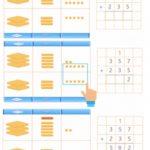Smartick is an online platform for children to master math in only 15 minutes a day

Content about adding and subtracting in elementary mathematics. Exercises, tutorials, and problems with adding and subtracting in elementary mathematics. Here you will find posts about adding and subtracting to make learning mathematics easier and more fun.

Oct09

## Strategies for Solving Incomplete Horizontal Additions and SubtractionsIn today’s post, we’re going to take a look at some strategies for solving problems that involve incomplete horizontal additions and subtractions, using examples to help us. Acquiring mental calculation strategies is not always easy, since there’s no defined “best method” for everyone, and it can be frustrating if you don’t pick it up quickly. […]

Sep25

## The Associative Property in Addition and Multiplication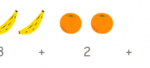Addition and multiplication both use the associative property, while subtraction and division do not. We’re going to to get up close with each situation to get a better idea. The associative property in Addition ♥ Addition indeed has the associative property. Whatever numbers a, b, and c may be, they always end up the same: […]

Sep01

## How to Solve a Subtraction Problem with Regrouping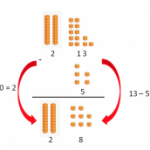Do you know how to solve a subtraction problem with regrouping? Do you know how regrouping is done and why? Today we are going to review how to subtract by regrouping and understand why it is done this way. So that we can picture it better, we are going to do a subtraction with regrouping […]

Jul24

## Round and Estimate Subtraction ProblemsIn this post, we are going to learn how to round and use estimation in subtraction problems. Mastering this skill is helpful in verifying that an exact answer is of the correct order of magnitude and also in determining whether an answer is reasonable or not. Round and Estimating Subtraction Problems in Tens Round the […]

Jul07

## Review Vertical Subtraction with Examples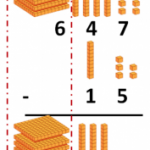In today’s post, we are going to review vertical subtraction. As always, we are going to review with examples that will help us to understand what vertical subtraction consists of and how to solve such problems. Before we begin, let’s review the material that we’ll use to subtract. We’ll use individual cubes to represent 1s. […]

Jun28

## Mental Math by Association

In this post, we are going to learn a mental math trick for adding and subtracting various numbers. It’s all about grouping the numbers that can give us exactly ten. In order to do so, we really need to pay attention to the numbers. Mental Math: Addition In order to add, we need to see […]

Jun26

## How to Add with Regrouping and Some Examples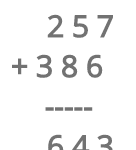In today’s post, we are going to learn how to add with regrouping.  Addition with regrouping consists of addition problems where the answer to the calculation is 10 or a bigger number. For example, 6 + 7 = 13 The steps to solve regrouping addition problems are the following: If the column being added doesn’t […]

Jun09

## Adding Strategy for Numbers That Are One or Two Values Apart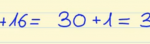In this post, we are going to learn a strategy for adding two consecutive numbers, or in other words, numbers that are one value apart. And a strategy for adding numbers that are two values apart. Adding Strategy: adding two consecutive numbers This strategy consists in first doubling the smaller number then adding one to […]

May26

## Learn How to Estimate a Sum with Examples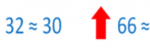In this post, we will learn to estimate a sum. Do you know what estimating a sum can help us with? When we have to do an operation mentally, often we do not need the exact result but an estimate of the outcome. Other times, after we have done an operation on paper and want to […]